Function Repository Resource:

# PursuitCurvePlot

Plot a predator-prey pursuit curve

Contributed by: Wolfram Staff (original content by Michael Trott)
 ResourceFunction["PursuitCurvePlot"][c,p,{t,t0,tf}] plots the pursuit curve of a predator starting at a point p, moving in the direction of the current position of the prey, following a path c from time t0 to time tf.

## Details and Options

ResourceFunction["PursuitCurvePlot"] has the same options as ParametricPlot and NDSolve, with the addition of "PursuitCurveDataFunction".
Possible settings for "PursuitCurveDataFunction" are:
 "DirectionOfMotion" plot vectors in the directions of motion of the predator and the prey "PredatorPreyVectorSet" plot a set of vectors from the predator to the prey as the curve evolves
The prey moves along a prescribed curve and the predator describes a pursuit curve, moving always in the direction of the prey with constant speed. If a third argument is not given, the predator and prey are taken to move at the same speed.
Let α=(x,y) and β=(f,g), and assume α is the pursuit curve of β. Then the statement that the predator and prey move at constant speed implies that x'2+y'2=k2(f'2+g'2). The statement that the predator always moves toward the current position of the prey is equivalent to x'(y-g)-y'(x-f)=0.
Integration stops when the distance between predator and prey is less than 10-3.
Pursuit curves were considered in general by Pierre Bouguer in 1732, and by George Boole.

## Examples

### Basic Examples (4)

Plot the pursuit curve for linear motion with unit speed:

 In:=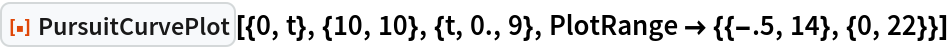Out=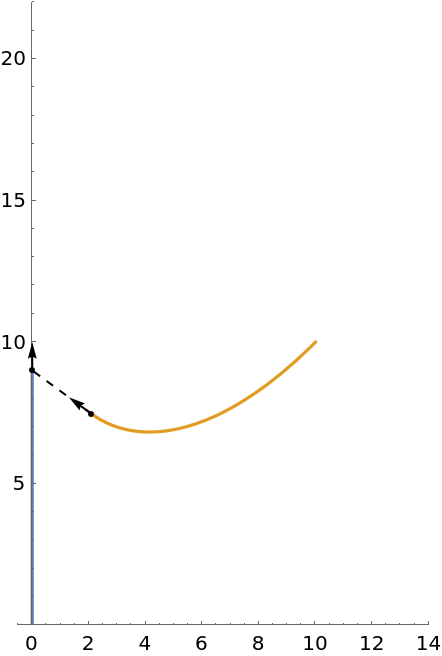Animate the pursuit curve over time:

 In:=Out=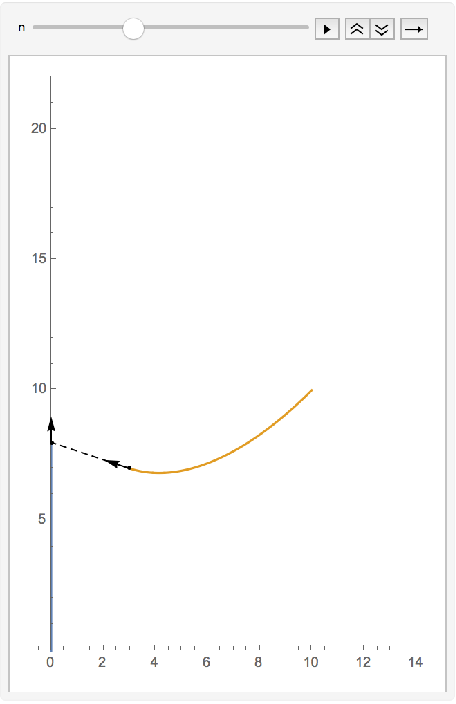Plot the pursuit curve for circular motion:

 In:=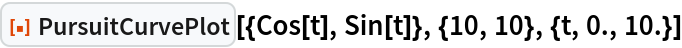Out=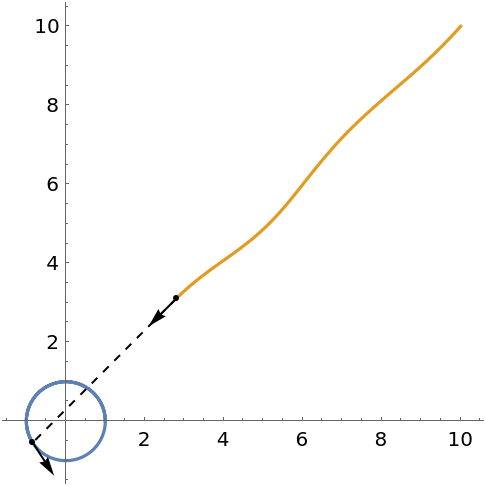Manipulate the curve evolution via the time parameter:

 In:=Out=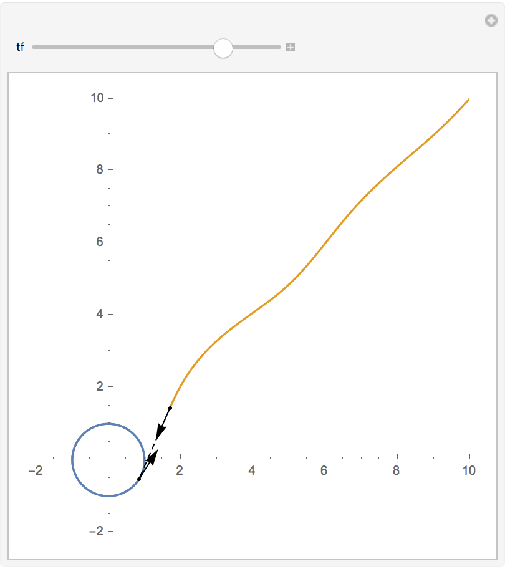A case where the predator never reaches the prey:

 In:=Out=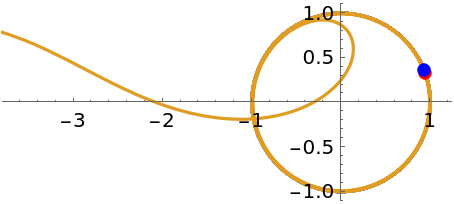Use a figure-eight prey curve:

 In:=Out=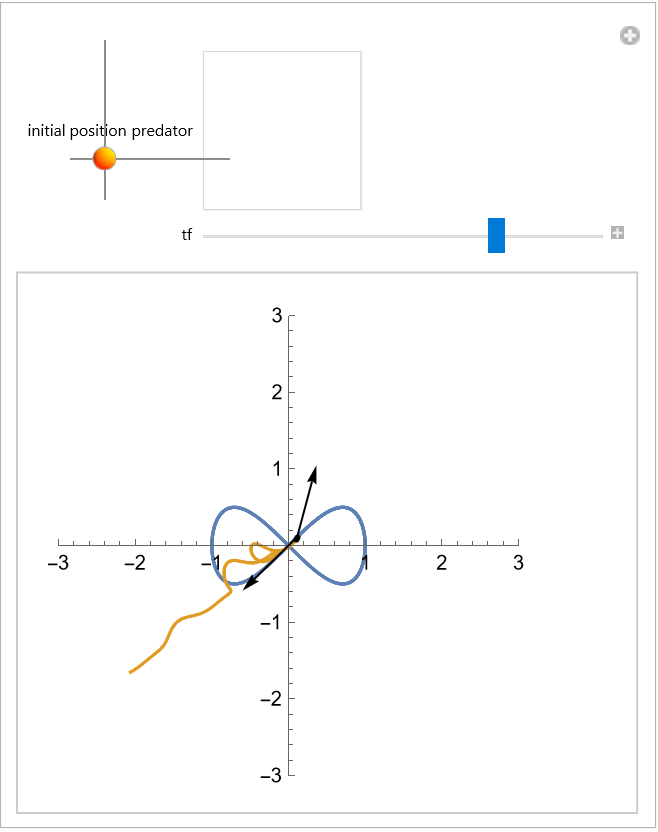### Options (3)

#### ColorFunction (1)

Use a gradient of colors for the curve:

 In:=Out=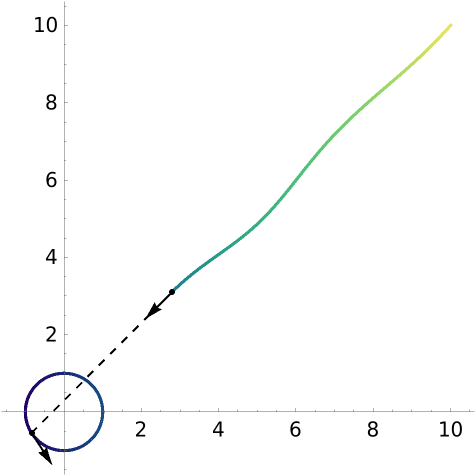#### PursuitCurveDataFunction (2)

Remove the vectors:

 In:=Out=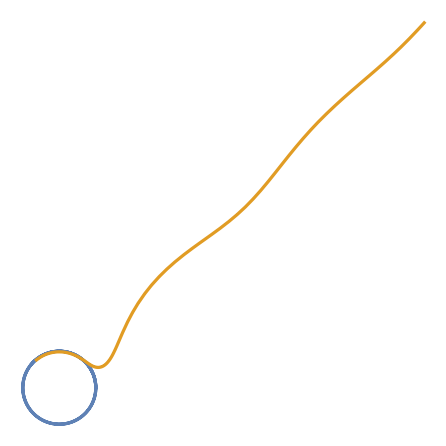Use a custom function to put points for current positions:

 In:=Out=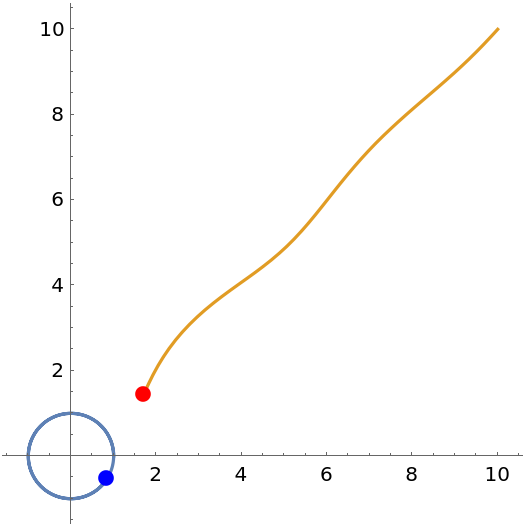### Neat Examples (2)

Include a plot of the distance between predator and prey:

 In:=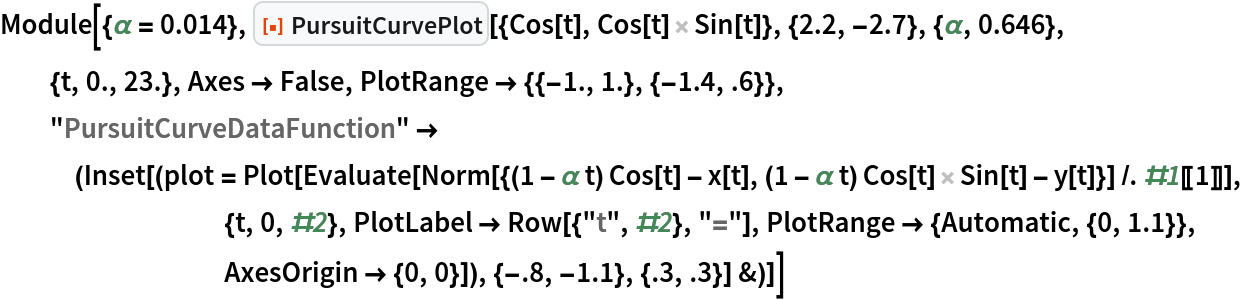Out=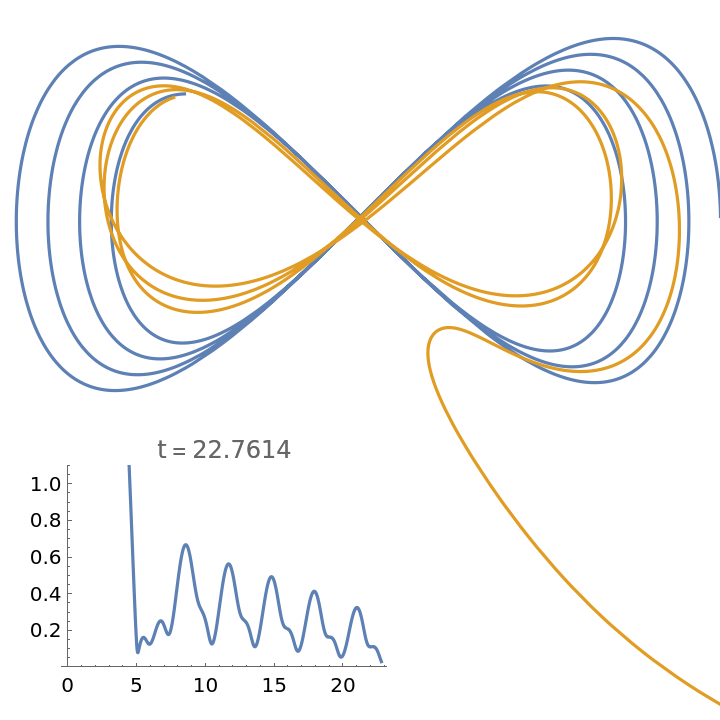Plot a set of vectors between the predator and the prey along the evolution of the curves:

 In:=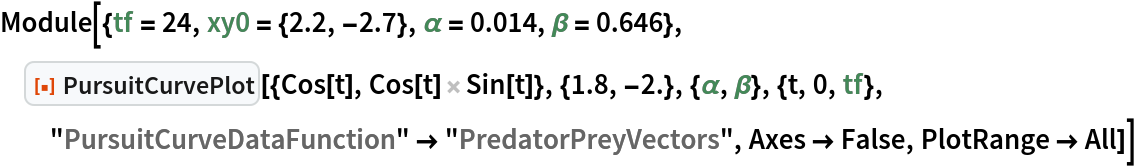Out=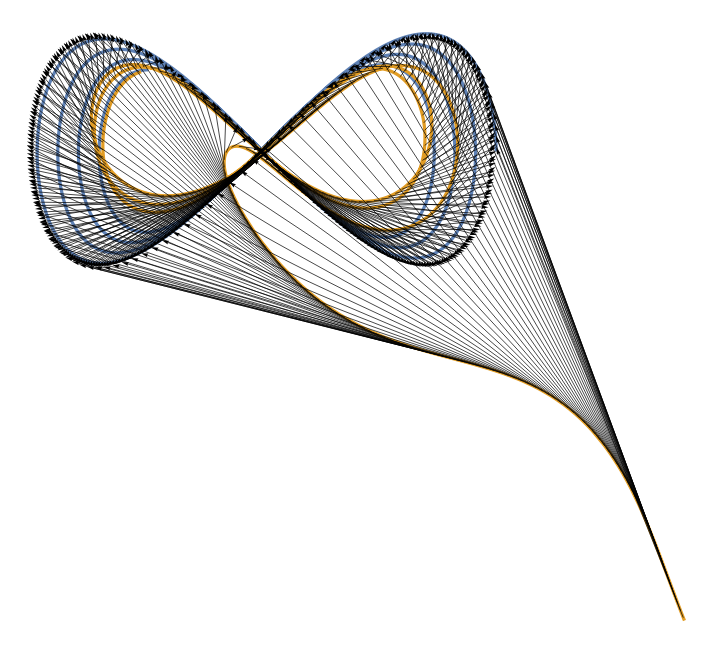Enrique Zeleny

## Version History

• 1.0.0 – 19 July 2021